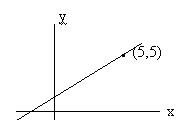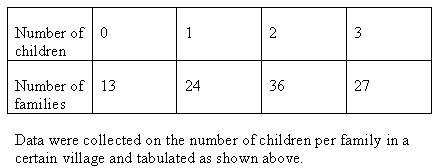50万+已关注

# SAT数学练习题整理11

2019-02-11 17:26:00来源：网络

新东方在线SAT频道为大家带来SAT数学练习题整理11一文，希望对大家SAT备考有所帮助。更多精彩尽请关注新东方在线SAT频道!

1. A straight fence is to be constructed from posts 6 inches wide and separated by lengths of chain 5 feet long. If a certain fence begins and ends with a post, which of the following could not be the length of the fence in feet? (12 inches = 1 foot)

A. 17

B. 28

C. 35

D. 39

E. 50

2. A circular logo is enlarged to fit the lid of a jar. The new diameter is 50 per cent larger than the original. By what percentage has the area of the logo increased?

A. 50

B. 80

C. 100

D. 125

E. 250

3. n denotes the number obtained when n is rounded to the nearest tenth. For example 4.31 = 4.3

0.089 - 1.135 =

A. 1.05

B. 1.04

C. -1.05

D. -1.0

E. -0.1

4. Half the people on a bus get off at each stop after the first, and no one gets on after the first stop. If only one person gets off at stop number 7, how many people got on at the first stop?

A. 128

B. 64

C. 32

D. 16

E. 85. The slope of the line passing through the point (5,5) is 5/6. All of the following points could be on the line except

A. (2.5, 2)

B. (11, 10)

C. (8, 7.5)

D. (-1, 0)

E. (-7, -5)

6. For a wall of a given material and of a fixed surface area, the rate of heat loss by conduction through the wall is inversely proportional to the thickness (T) of the wall, and directly proportional to the temperature difference (d) across the wall. The rate of heat loss could be given by which of the following expressions?

A. 2d/T

B. 2 T/d

C. 2d + T

D. 2(d + T)

E. T/d7. The average (mean) number of children per family in the village is approximately

A. 2.4

B. 2.0

C. 1.8

D. 1.5

E. 1.0

8. Refer to the table provided in the previous question.

The difference between the median and the mode of the number of children per family is

A. 2.5

B. 2.0

C. 1.5

D. 1.0

E. 0

9. If x is an integer, which of the following could be x3?

I 1.25 x 1017

II 12.5 x 10-12

III 0.125 x 10-12

A. I only

B. II only

C. I and II only

D. I and III only

E. II and III only

10. At what point does the graph of 5x + 4y = 12 intersect the y-axis?

A. (0, 3)

B. (0, -3)

C. (3, 0)

D. (5, 0)

E. (0, -5)

Explanation:

The fence will consist of one more post than there are chains. (e.g. P-c-P-c-P).

Therefore, a total length has to be a multiple of the length of the chain plus one post (5.5) plus one post extra.We have length = (5.5n + 0.5), where n can be any positive whole number. If n= 3, length =17; if n= 5, length = 28, etc.but there is no whole number that can give 35. hence C is the correct answer.

Explanation:

If the diameter of the old lid is 100, the diameter of the new lid will be 150.

This gives a ratio of 2 : 3

The areas of the lids will be in the ratio (2)2 : (3)2 . or 4 : 9

This represents an increase in area of 5units for every 4 units

5/4 is equivalent to 125 percent.

Explanation:

0.089 to the nearest tenth = 0.1

1.135 to the nearest tenth = 1.1

0.1 - 1.1 = -1

Explanation:

Work backwards. If one person got off at stop 7, as the bus approached the stop there must have been two people on board. As it approached the previous stop (no. 6) there were four on board. Approaching stop 5, there were 8. Approaching stop 4, there were 16 on board. Approaching stop 3, there were 32. Approaching stop 2, there were 64. As stop 2 was the first stop at which people got off there must have been a total of 64 people who boarded at the first stop. (We are assuming that no people could have been on the bus before the first official stop.)

Explanation:

If we consider the triangle bounded by the x axis, the line, and the perpendicular to the x-axis passing through (5,5), then, since the slope (5/6) is given by the y-side (5 units) of the triangle divided by the x side, the x side is 6. This means the intercept on the x-axis is at (-1, 0). Now consider other triangles bounded by the line, the x-axis and the perpendicular to the points given in the answer choices, and check that they give a slope of 5/6. For example, in (A) the x side of the triangle will be 2.5 + 1 units long, and the y side will be 2. This does not give a slope of 5/6. (You can use the formula y2 - y1 / x2 - x1 to find the slope. For this use the intercept on the x axis for x1 and y1)

Explanation:

Rate (R) is directly proportional to d. We write this as R = k.d, where k is a constant.

Rate is inversely proportional to T. We write this as R = k. 1/T, where k is a constant.

In the combined expression, d must appear in the numerator and T in the denominator. This is the case in A.

Explanation:

To find the average we need to find the total number of children (1x24 + 2x36 + 27x3) = 24 + 72 + 81 = 177, and divide by the total number of families (13 + 24 + 36 + 27) = 100. This give 177/100 = 1.77 which is approx. 1.8

Explanation:

The modal value for the number of children per family is the number that occurs most. Since the largest number of families have 2 children, the mode is 2.

The median number is also 2 because we have 100 families and if we write down the number of children per family in ascending order we would write 13 zeros, followed by 24 ones followed by 36 twos etc… and the median will be the average of the numbers that occur at positions 50 and 51 in this list. These numbers will both be 2.

Explanation:

We can shift the decimal points so that we get 125 and a power of ten. 125 is a cube. If the power to which ten is raised is divisible by 3, then that is also a cube.

For statement I we get 125 x 1015 (We have multiplied the 1.25 by 100 and so we must divide 1017 by 100 which means we subtract 2 from the power). For statement II we get 125 x 10-13, and for III we get 125 x 10-15. But in the case of III the answer is a negative power making it a fraction NOT an integer.Only in the case of I is the power divisible by three.

Explanation:

Rearrange the equation to fit the form y = mx + c, where c is the intercept on the y-axis. At this point x = 0.

4y = 12 – 5x; y = -5/4 x + 12/4; 12/4 = c = 3

以上就为大家整理的“SAT数学练习题整理11”，更多精彩内容，请关注新东方在线SAT频道。

SATII精讲班
SAT名师一对一

SAT考生必修课
SAT考生，高效提分，安心之选

• 课时 : 91琚晋蓉张霄

￥2680

18人 已报到

免费试听

• 1
• 2
• 3
• 4
• 5
• 6
• 7
• 8
• 9
• 10